By Pat Sagsveen

Contributed By Digi-Key Electronics

Today’s world is littered with digital and analog signals. While these signals behave differently, they are often both used to help achieve a larger goal. Imagine the engineer tasked with controlling an HVAC unit. If planning to use any kind of microcontroller or microprocessor, it will be necessary to be able to read an analog temperature which has an infinite amount of values and convert that into a binary representation laid out in discrete steps. This binary representation of the analog value will then be processed by the microcontroller or microprocessor. This data will be used to help execute a process by the HVAC unit to help maintain a stable environment. When dealing with an analog value that needs to be processed by a digital system, an analog-to-digital converter (ADC) will be imperative. The same theory can be applied backwards to a digital signal that needs to be converted into an analog signal. Streaming a song online involves a few different steps that use the conversion of digital signals to analog signals. The signal that the host device receives from the server will be a binary representation of the original analog signal. The audible response from this binary data would be unintelligible to the listener. The original signal was analog so the final representation of that will also need to be analog. This problem is solved by the use of the digital-to-analog converter (DAC). This type of device takes a binary code that could have been encoded by an analog to digital converter, and turns it back into an analog voltage.

Converting signals from analog to digital or digital to analog is an unavoidable task for today's engineer. There are many different kinds of analog to digital converters and digital to analog converters. While these differ in their architecture, they all work to achieve a similar end. Since digital signal processing cannot be done with analog values, it would be analogous to a French speaking person trying to speak with a German speaking person. It would not work without a translator. ADC and DAC devices can help to act like that translator. When an ADC sees an analog voltage, its job is to turn the analog voltage into a binary code at a given period of time. This means the ADC will sample the analog voltage at an instant, and then it determines what the value would be in binary on the output side of the ADC. The amount of samples that the device takes every second will be called out on its documentation. An example of this would be the MAX1118EKA+T from Maxim Integrated. This device has a 100 kHz sampling rate so it can sample the analog voltage on its input side 100,000 times per second. By being able to take that many samples in a second, it is possible to accurately log what the analog voltage looked like by using a binary interpretation. Sometimes the sample rate of an ADC is not high enough to accurately recreate its input which causes aliasing. This is where signals start to be indistinguishable from one another, or aliases of one another. Imagine a video camera that can take 24 frames per second when recording. For most applications this will be fine; however, if trying to view something that is moving very fast, this can distort the image. Recall the effect of watching a television on a recording in the late nineties. The image on the television would be flickering. This is because the refresh rate on the television itself is much faster than the recording can capture at its given frames per second. The image would be distorted because the video is actually a succession of pictures. There is more happening between each picture than is actually being represented on the video. The same kind of effect can happen with an ADC. To avoid this, it is pertinent to make sure the sample rate is at least two times higher than the highest frequency that needs to be transmitted. This is referred to as the Nyquist rate.

Having a greater sample rate will allow the device to be more accurate, but this is not the only way to control accuracy. Since this is converting an analog signal into a binary code, there will be a finite number of discrete steps that can be used to represent the voltage at a point in time.  The number of bits that can be used to represent this number is the resolution. The higher the resolution is for the ADC, the more discrete steps that can be taken by it. To further detail this it is important to understand how to determine how many steps an ADC can make. An ADC has a binary output that is representative of the supply voltage. If the supply voltage is 10 V and there is an 8-bit ADC, then there is a possibility of 256 steps. To determine the resolution use the equation 2n. The “2” is constant and the N is how many bits there are. By taking 28 we end up with 256 steps. Using 256 steps with a 10 V supply means that each step will be 39.0625 mV. There will be a different binary code for each of these steps. If one was to run through all the possible input options on an ADC starting from lowest and working to highest, they would see the outline of a staircase. This staircase is the transfer function of the ADC. Figure 1 illustrates the transfer using a 3-bit ADC with a 2 V reference.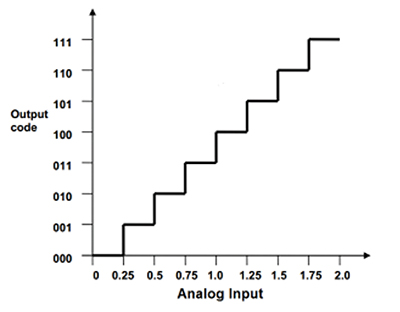Figure 1: Transfer function of a 3-bit ADC with a 2 V reference. (Courtesy of Microchip Technology)

Since there are three bits, it is possible to calculate the total number of steps by using the 2n equation as follows: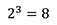As evident in Figure 1, there are eight steps between 000 and 111. Every step will move up one LSB.

As previously mentioned, there are several different kinds of ADC architectures. The three most popular ADC architectures are Successive Approximation Registers (SAR), Delta-Sigma (∆∑), and Pipeline converters. Each of these will convert an analog signal into a digital output, but there are slight differences in how this is done. The SAR will sample an analog input and hold it, turn it into a digital signal, then pass it off. Delta-Sigma converters will average the sample over the time it takes to convert it into a digital signal. Pipelined converters divide the conversion into different stages allowing for very fast conversion speeds. Each of these will have positives and negatives. The SAR architecture will be easy to use, typically use lower power, and have low latency time with high accuracy. The Sigma-Delta will have a very high resolution and high stability at low power and low cost; however, it will work at much lower speeds than the SAR and Pipeline architectures. The Pipeline ADC will work at the higher speeds and higher bandwidth than the previous examples, but will have a lower resolution and require more power to run.

## Successive approximation registers

Successive Approximation Registers are the most popular types of ADC. They will often have an I2C or SPI interface, but sometimes there will be a parallel output. To help process the analog signal SAR ADCs will have a sample and hold to try to keep the signal constant. There is a comparator that measures the analog input against an internal DAC. This DAC will be set to ½ of its potential voltage at this point. If the input is higher than the DAC, the comparator will output a 1 to be stored in the MSB in the “successive approximation register”. After this the DAC will be set to ¼ of its potential voltage and the process repeats. The next value for the DAC would be 1/8 then 1/16 and so on until all of the bits had been loaded into the register. There is an analogy that helps to illustrate this which involves successively adding or not adding weights to see how much something else weights. Figure 2 below illustrates this point.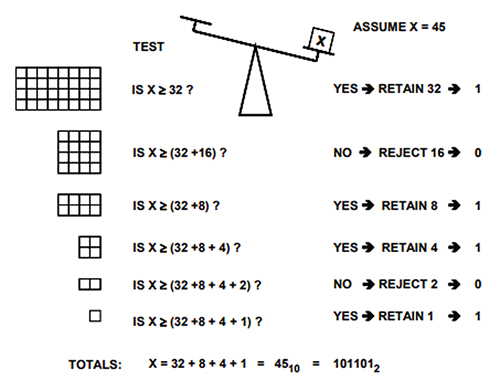Figure 2: Illustrative analogy of how a successive approximation ADC obtains a digital value from an analog value. (Courtesy of Analog Devices)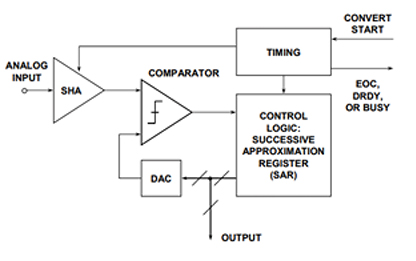Figure 3: Successive approximation ADC functional block diagram. (Courtesy of Analog Devices)

An example of a SAR ADC is the ADS7886SDBVT from Texas Instruments. This is a 12-bit ADC meaning that it can take 4,096 steps between 0 V and supply voltage. The sampling rate is 1 MHz, which means this looks at its input one million times per second. Since ADC performance should be tested before being implemented into a finished product, often manufacturers will make an evaluation tool to help in testing. The ADS7886SDBVT has such an evaluation tool, it is the ADS7886EVM. This makes testing much easier because there is already a board laid out with all of the passive components that will be necessary to functionally test the ADC.

## Sigma Delta ∆∑

Sigma Delta converters are very popular choices for anything that needs high resolution and precision. Audio recording would be a good example of an application for a Sigma Delta converter. Sigma Delta converters need to oversample their inputs. The Nyquist rate is not as relevant when discussing Sigma Delta converters, they will work optimally when the sample rate is about 20 times higher than the highest frequency to be sampled. The output of the Sigma Delta converter is fed into a digital filter and decimator that processes the bit stream to produce the final output. Often there will be a serial interface between the digital filter and output. An example of a Sigma Delta ADC would be the AD7175-2BRUZ from Analog Devices, Inc. Figure 4 shows the “Functional Block Diagram” of the AD7175-2BRUZ.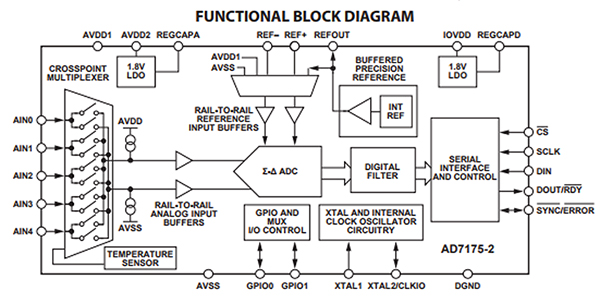Figure 4: Functional block diagram of the Analog Devices AD7175-2BRUZ Sigma-Delta ADC. (Courtesy of Analog Devices)

This Sigma Delta ADC has a 24-bit resolution and a sampling rate of 250 kHz. This will have a potential of up to 16,777,216 steps. This means there is much higher resolution than the previous example; however, about ¼ of the sampling rate. Like the previous example, the AD7175-2BRUZ also has an evaluation board for testing purposes. This board is the EVAL-AD7175-2SDZ and provides an easy way to evaluate the ADC.

## Pipelined

The pipelined ADC will be the fastest. An example from “ADC Architectures V: Pipelined Subranging ADCs” helps to illustrate this using a 6-bit pipelined ADC. The sample and hold is like the SAR, but directly after the sample and hold there will be a 3-bit sub-ADC flash converter which digitizes the signal. The 3-bit conversion will be for the 3 most significant bits. This will be converted back to an analog signal by using a sub-DAC. This output will be subtracted from the sample and hold output, amplified, then sent back into the second stage 3-bit sub-ADC for the three remaining least significant bits. Figure 5 has a block diagram that illustrates this point.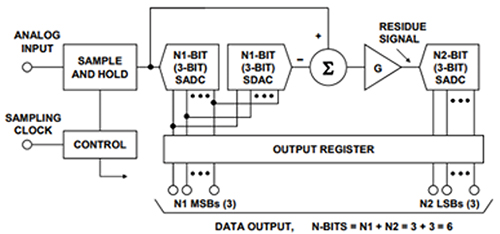Figure 5: Pipelined subranging ADC functional block diagram. (Courtesy of Analog Devices)

An example of a pipelined ADC would be the ADC10080CIMT/NOPB from Texas Instruments. This is a 10-bit ADC, so it has a potential of 1,024 steps and can convert 80 Megasamples per second. Applications for this device would be ultrasound and imaging, instrumentation, data acquisition systems, or just about anything that involves fast conversion. Looking at the ADC10080CIMT/NOPB block diagram, one can see how it is set up like described above. Figure 6 has the block diagram taken from the datasheet of the ADC1008CIMT/NOPB.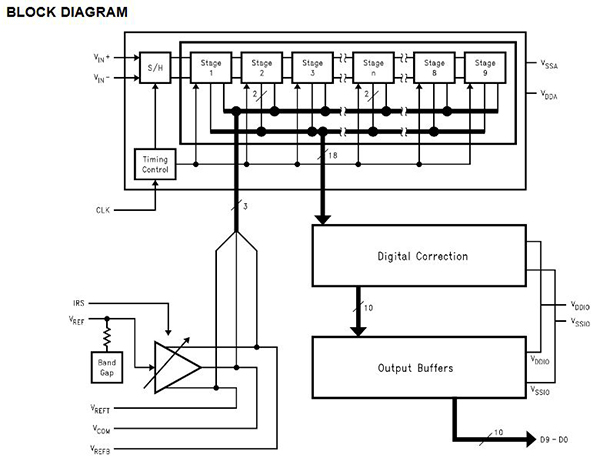Figure 6: The Texas Instruments’ ADC10080CIMT/NOPB functional block diagram. (Courtesy of Texas Instruments)

## Binary weighted DAC

Up to this point, this article has focused on the ADC side of data conversion, but this is only half of the battle. It is often necessary to convert binary data back into an analog signal. This is where the digital-to-analog converter comes in. Often the first type of DAC that is taught in school is the Binary-Weighted DAC. This involves using a system of resistors whose outputs all meet at the same summing resistor. The more significant bits will output more current than the less significant bits. This is done by creating an inversely proportional resistive network. Since each binary bit of the digital code will have the same voltage value, by using inversely proportional resistors on each bit, the greater bits will allow more current to flow through them. This particular method of conversion is not very popular anymore because of easier methods used today; however, it is a very good starting point for describing how DACs work. The largest difficulty with this method is finding different level resistors that will work together. The tolerance would need to be incredibly tight and it is just easier to find similar valued resistors instead of several different values like the Binary Weighted method uses. Figure 7 illustrates this architecture by using a schematic of a Binary Weighted resistor network.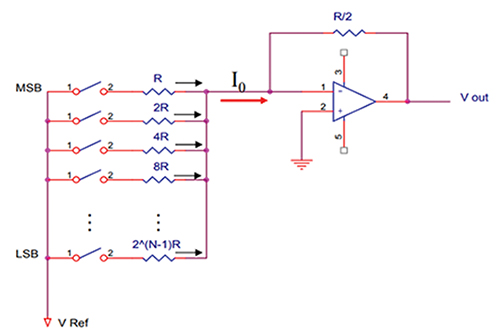Figure 7: Schematic representation of a binary weighted register network. (Courtesy of Georgia Institute of Technology)

## String DAC

Another popular architecture for DACs is the String DAC. This is the simplest architecture, but also least linear, and is sometimes called the Kelvin divider. This will have a string of equal valued resistors in series. The string will have a reference voltage on top, a high impedance resistor before the string, and each node will have a switch that is dedicated to a binary code that will close when that code is read by the device. This allows for an analog voltage value to be used which is dependent upon the binary input. Figure 8 comes from a video titled “What is a String DAC?” from Texas Instruments. The code 010 is chosen for the decimal value of 2. The switch at 010 is now closed allowing the DC voltage at that node to be passed to the summing amp.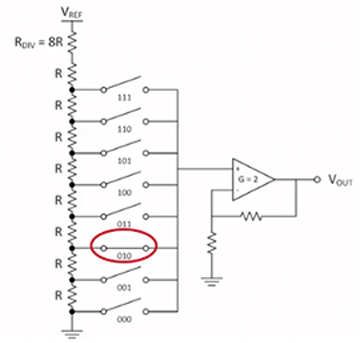Figure 8: String DAC schematic representation. (Courtesy of Texas Instruments)

The string DACs are easy to produce because they use the same value resistor for every part of the string (minus the high impedance resistor).  An example of a String DAC would be the AD5683RBRMZ from Analog Devices Inc. This is a 16-bit String DAC that works with SPI. Figure 9 shows a functional block diagram of this device and Figure 10 shows the resistive divider. This product also has an evaluation tool, the EVAL-AD5683RSDZ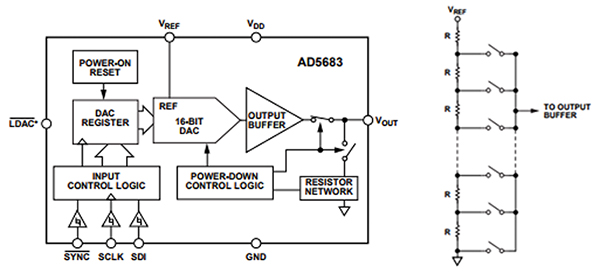Figures 9 & 10: Functional block diagram of the Analog Devices AD5683RBRMZ (left) and its resistor divider network (right). (Courtesy of Analog Devices)

## R-2R

The R-2R network is a very common DAC architecture. This uses only two values of resistors, their values will not matter as long as 2R is twice as large as R. This makes R-2R DACs very scalable. Regardless of how many bits the DAC is, there will still only be a need for two values of resistors. Figure 11 shows a 4-bit R-2R ladder network.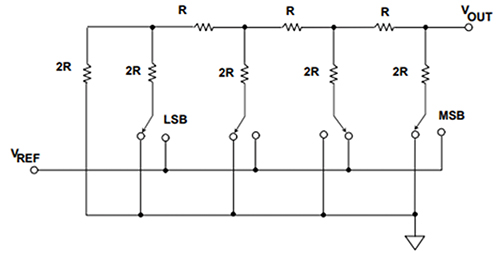Figure 11: Schematic representation of an R-2R DAC. (Courtesy of Analog Devices)

This divider network uses Thevenin’s theorem to ultimately get the Thevenin’s equivalent resistance for the whole network of “R”. Every stage will allow double the voltage as the last stage. In this example, if the Vref was 5 V, then the furthest left stage for the LSB would only be able to produce 0.3125 V. Moving from left to right, the proportional voltage output would be: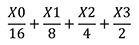This is representative of all of the digital inputs, X0 is the LSB and X3 is the MSB. Using a 5 V reference would give us: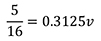By the same logic, to find what the analog voltage would be for the most significant bit it would be possible to take the following: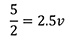If there was a binary input of 1111, the output would not equal 5 V. Recall the discussion on resolution earlier. If there is a 4-bit DAC, then there can be 16 steps. This is because 24 would allow for 16 steps. The LSB is at 0.3125 V, if one was to multiply 0.3125 x 16 they would arrive at 5 for an answer. This is misleading because one of the steps will involve a 0 V ground to represent binary 0000. This means that the highest voltage that can be achieved is the supply voltage minus one LSB voltage. This leaves us with 4.6875 V.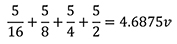An example of an R-2R DAC would be the DAC8734SPFB from Texas Instruments. This is a 16-bit converter which goes to show how scalable these devices are. The layout used for the R-2R network in this is very similar to what was described above. Figure 12 shows the R-2R network for the DAC8734SPFB. There is also an evaluation tool for the DAC8734SPFB, the DAC8734EVM.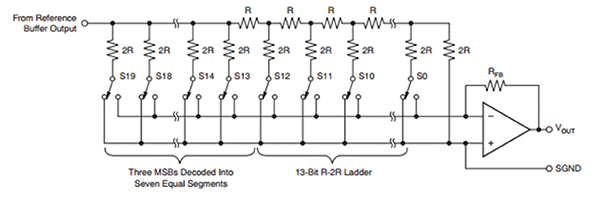Figure 12: Texas Instruments’ DAC8734SPFB R-2R DAC schematic representation. (Courtesy of Texas Instruments)

There are many other kinds of ADC/DAC architectures, but this article only covers some of the more popular architectures. These devices are necessary in today’s world of digital signal processing. Without ADC/DAC devices it would not be possible to integrate an analog output into any kind of digital signal processing or vice versa. It is easy for the layperson to take this for granted, but for the engineer tasked with connecting analog components up to a digital system, this is one of the most important steps that needs to be considered. When thinking of Analog to Digital, recall the analogy of the two people speaking different languages. If those people did not have a translator they would not be able to communicate with one another. Without properly utilizing ADC/DAC devices, a device would have to use strictly analog or strictly digital. ADC/DAC devices are a great handshake between both worlds.

## Resources

1. AVR127: Understanding ADC Parameters”. May. 2016.
2. Choose the right A/D converter for your application”. Retrieved 8 May. 2017.
7. Kester, Walt. “DAC Interface Fundamentals”. Oct. 2008.
8. Kester, Walt. “Basic DAC Architectures II: Binary DACs”. Oct. 2008.
9. Lee, J., Jeelani K., Beckwith, J. “Digital to Analog Converter”. Retrieved 8 May. 2017
10. Poole, Matt. “What is a String DAC?”. April 4, 2016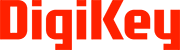Disclaimer: The opinions, beliefs, and viewpoints expressed by the various authors and/or forum participants on this website do not necessarily reflect the opinions, beliefs, and viewpoints of Digi-Key Electronics or official policies of Digi-Key Electronics.